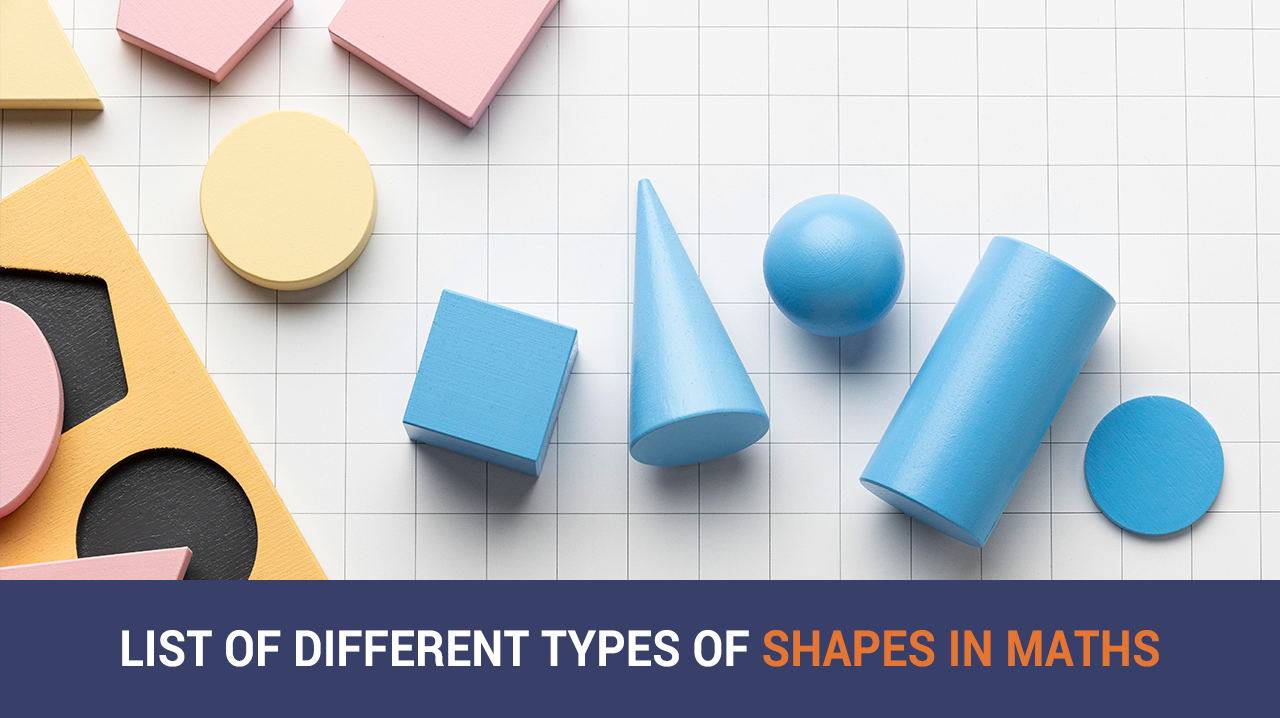# List Of Different Types Of Shapes In Maths | ImagesMathematics, geometry, and shapes have always been fascinating to learn and think about. In our everyday lives, we see the application of different types of shapes, right from the dosa we eat to the tennis ball we play with.   There are many different kinds of shapes, each distinct from the other in one way or the other. Each type of shape is used in our daily lives and works in tandem with mathematics. Concepts like trigonometry can determine the heights of buildings and other structures without any tools. Finding the volume of a water tank is, again, a combination of basic mathematical operations and shapes.   Knowing different types of shapes might seem trivial, but it is helpful to know their applications. In this article, we discuss the types of shapes in maths and understand how they are applied in our daily lives.

## What are Shapes in Maths

Before we know the various kinds of shapes, it is crucial to understand what shapes mean in mathematics and general. A shape is any closed figure of angles, line segments, points, and sometimes circles. Forms can be two-dimensional or three-dimensional.  Let us now understand about different shapes and their names.

## List of Different Types of Shapes In Maths

### Circle:A round shape that is equally shaped. A circle as a shape has many sub-sets and has innumerable applications. The bottle cap is a circle, and so is the tyre of a bicycle.

### Triangle:As the name suggests, this shape has three angles. Triangles are of many kinds, each having a different distribution of curves and are generally pointy. A slice of pizza is the most common daily object with a triangle shape.

### Square:Squares are the most common shapes. These shapes in maths have various applications, from a flooring tile to a container box. A square has four sides, and each size is equal. A square also has four angles.

### Rectangle:Hailing from the square family, the rectangle also has four sides. However, in this type of shape, the opposite sides are equal. The most common rectangle object is a Television.

### Pentagon:This shape has five sides. A pentagon is usually drawn with two parallel lines as a roof, two lines on the side and one on the bottom.

### Hexagon:A hexagon is a six-sided shape. A hexagon can be observed in honeycombs and air filters. Hexagon has many variants, making these types of shapes very unique.

### Octagon:An octagon is an eight-sided polygon. This shape is ornamental and can be found in jewelry and decorative items.

### Ellipse:An ellipse is a circle that is bulged on the sides. The bulge makes the shape not a perfect process, which extends the size of the shape. The planet Earth is elliptical, and so are convex lenses.

### Parallelogram:A parallelogram is close to a rectangle where the opposite sides are equal; however, the interior opposite angles are equal, unlike a rectangle where all four angles are equal.

### Rhombus:This shape is similar to a square as all its four sides are of equal lengths. A square has all its angles equal to 90 degrees, but in a rhombus, only the opposite angles are equal.

### Trapezoid:This unique shape is a quadrilateral and is two-dimensional. In this shape, there are two parallel lines. A trapezoid can have different lengths on all four sides.

### Kite:This shape also has four sides where only the opposite sides are equal, and these sides are adjacent to each other. The recreational object kite derives its name from this shape.

### Regular Polygon:A regular polygon is a shape with equal sides and interior angles. Examples of regular polygons are square and equilateral triangles.

### Irregular Polygon:In this shape, not all sides are equal, or not all angles are equal. Examples of an irregular polygon are a rectangle, an isosceles triangle, and a rhombus.

### Cylinder:A cylinder is a three-dimensional shape. This shape has two circles on the opposite sides as its base. The most common application of a cylinder is a water tank or a CNG Cylinder.

### Sphere:This is a solid, three-dimensional shape. It is a solid version of a circle, and its most famous application is as a ball.

### Cube:A cube has six square faces and is a three-dimensional shape. The most common application of this shape are playing dice.

### Cuboid:This shape is a three-dimensional rectangle. This shape has six rectangular faces. The common use of a cuboid is a pencil box.

### Cone:A cone is a solid, three-dimensional shape. This shape starts from a flat, broader base to a narrow point. Ice cream cones apply the concept of this shape.

### Pyramid:A pyramid is a three-dimensional shape with a polygonal base. The sides are flat triangles.

The list contains different types of shapes and their names. The world of mathematics is vast and unique, making shapes enjoyable.

## 1. What shape has all sides of equal length and all angles of 90 degrees?## 2. Which shape has three sides and three angles?## 3. What is a polygon with five sides called?## 4. Which shape has no straight sides and all its points are equidistant from the center?